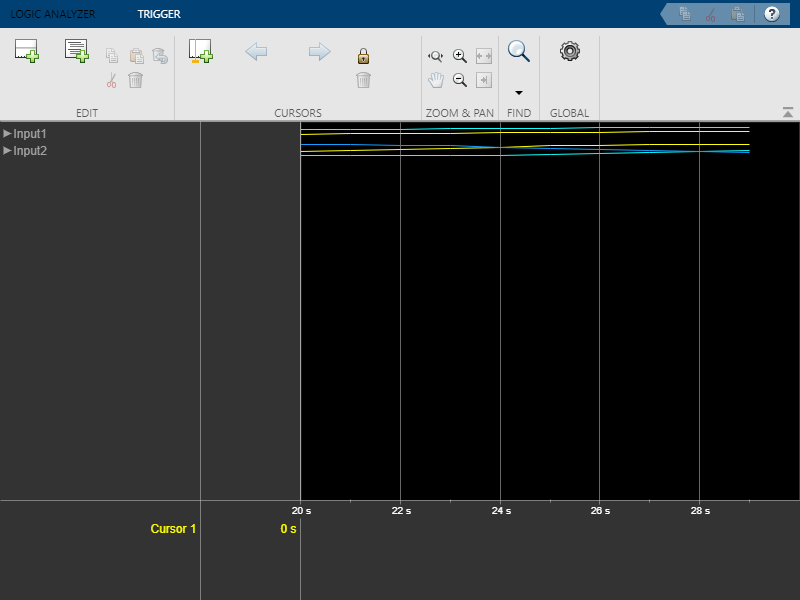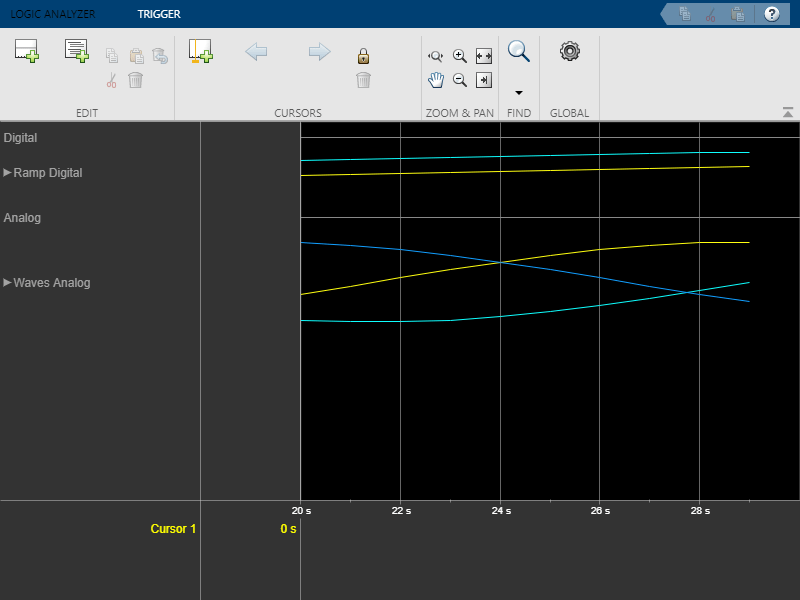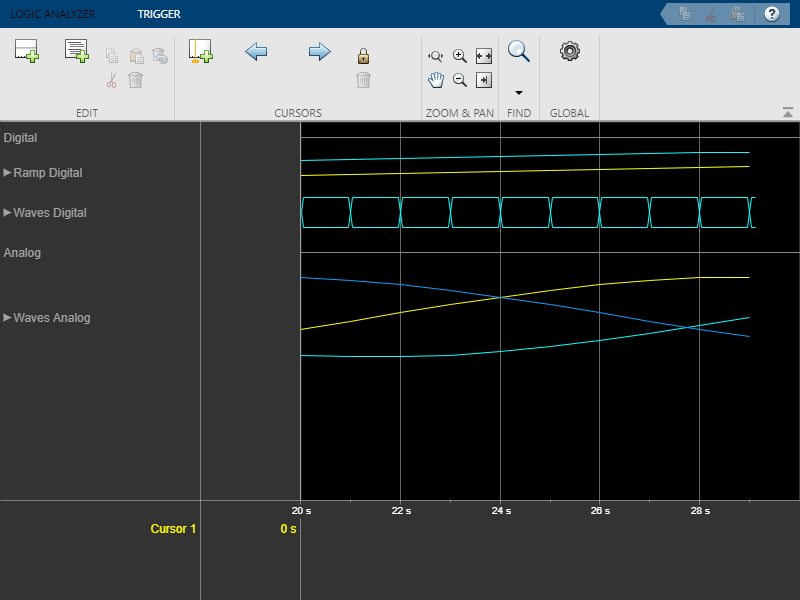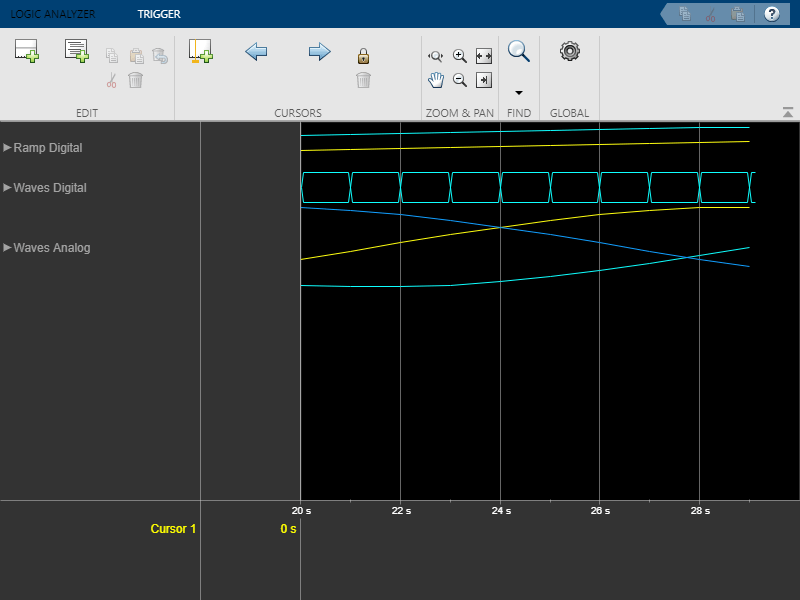## Syntax

``dividerTag = addDivider(scope)``
``dividerTag = addDivider(scope,Name,Value)``

## Description

example

````dividerTag = addDivider(scope)` adds a divider to the display. A tag value is returned, which can be used to modify and delete the divider. `dividerTag = addDivider(scope,Name,Value)` sets properties using one or more name-value pairs. Enclose each property name in single quotes.```

## Examples

collapse all

Use functions to construct and manipulate a dsp.LogicAnalyzer System object.

Display Waves on Logic Analyzer scope.

```scope = dsp.LogicAnalyzer('NumInputPorts',2); stop = 30; for count = 1:stop sinValVec = sin(count/stop*2*pi); cosValVec = cos(count/stop*2*pi); cosValVecOffset = cos((count+10)/stop*2*pi); scope([count (count-(stop/2))],[sinValVec cosValVec cosValVecOffset]) end```Reorganize Display

```digitalDividerTag = addDivider(scope,'Name','Digital','Height',20); analogDividerTag = addDivider(scope,'Name','Analog','Height',40); tags = getDisplayChannelTags(scope); modifyDisplayChannel(scope,tags{1},'InputChannel',1,... 'Name','Ramp Digital','Height',40); modifyDisplayChannel(scope,tags{2},'InputChannel',2,... 'Name','Waves Analog','Format','Analog','Height',80); moveDisplayChannel(scope,digitalDividerTag,'DisplayChannel',1) moveDisplayChannel(scope,tags{2},'DisplayChannel',length(tags)) show(scope)```Duplicate Wave and Check Information

```addWave(scope,'InputChannel',2,'Name','Waves Digital','Format','Digital',... 'Height',30,'DisplayChannel',3);```Remove Dividers

```deleteDisplayChannel(scope,digitalDividerTag) deleteDisplayChannel(scope,analogDividerTag)```Clear variables

`clear analogDividerTag cosValVec cosValVecOffset count digitalDividerTag duplicateWave scope sinValVec stop tags`

## Input Arguments

collapse all

`dsp.LogicAnalyzer` object to which you want to add a divider.

Example: `addDivider(scope)` adds a divider with the default characteristics.

### Name-Value Pair Arguments

Specify optional comma-separated pairs of `Name,Value` arguments. `Name` is the argument name and `Value` is the corresponding value. `Name` must appear inside quotes. You can specify several name and value pair arguments in any order as `Name1,Value1,...,NameN,ValueN`.

Example: `'DisplayChannel',2,'Name','MyDivider'` specifies that a divider should be added to display channel 2 and named “MyDivider”.

Specify as a scalar numeric value the display channel that shows this divider. By default, the divider is added to the end of the display.

Example: `'DisplayChannel',2`

Data Types: `double` | `single` | `uint8` | `uint16` | `uint32` | `uint64` | `int8` | `int16` | `int32` | `int64`

Specify, in pixels, the height of the divider as a scalar integer in the range 8-200. If you choose 0, the value of the `DisplayChannelHeight` property in the Logic Analyzer is used.

Example: `'Height',2`

Data Types: `double`

Specify the name that you would like to set for the new divider.

Example: `'Name','MyDivider'`

Data Types: `char` | `string`

## Output Arguments

collapse all

A tag for the newly added divider. Use the tag name to modify and delete the divider.